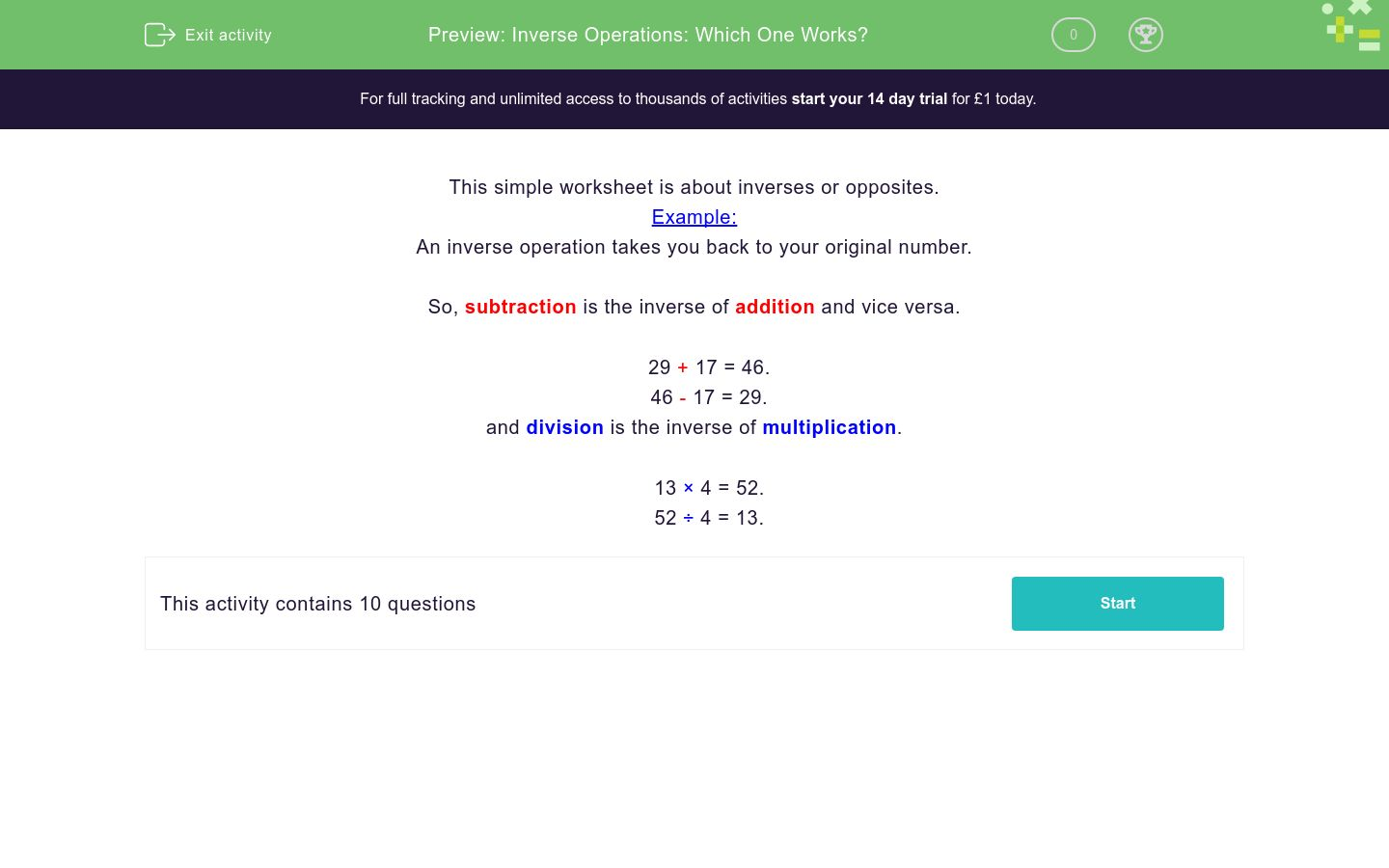# Inverse Operations: Which One Works?

In this worksheet, students choose the correct inverse operation to return to the original number in a calculation.Key stage:  KS 3

Curriculum topic:   Number

Curriculum subtopic:   Use Relationships Between Operations

Difficulty level:### QUESTION 1 of 10

This simple worksheet is about inverses or opposites.

Example:

An inverse operation takes you back to your original number.

So, subtraction is the inverse of addition and vice versa.

29 + 17 = 46.
46 - 17 = 29.

and division is the inverse of multiplication.

13 × 4 = 52.
52 ÷ 4 = 13.

Using your calculator, decide which single operation would take you back to the original number.

131 − 119 = 12

Inverse operation: _______

+

-

x

÷

Using your calculator, decide which single operation would take you back to the original number.

262 × 647 = 169514

Inverse operation: _______

+

-

x

÷

Using your calculator, decide which single operation would take you back to the original number.

805 − 491 = 314

Inverse operation: _______

+

-

x

÷

Using your calculator, decide which single operation would take you back to the original number.

593 × 952 = 564536

Inverse operation: _______

+

-

x

÷

Using your calculator, decide which single operation would take you back to the original number.

437 × 477 = 208449

Inverse operation: _______

+

-

x

÷

Using your calculator, decide what single operation would take you back to our original number.

4577 ÷ 199 = 23

Inverse operation: _______

+

-

x

÷

Using your calculator, decide which single operation would take you back to the original number.

930 + 549 = 1479

Inverse operation: _______

+

-

x

÷

Using your calculator, decide which single operation would take you back to the original number.

311 × 995 = 309445

Inverse operation: _______

+

-

x

÷

Using your calculator, decide which single operation would take you back to the original number.

840 + 290 = 1130

Inverse operation: _______

+

-

x

÷

Using your calculator, decide which single operation would take you back to the original number.

11520 ÷ 45 = 256

Inverse operation: _______

+

-

x

÷

• Question 1

Using your calculator, decide which single operation would take you back to the original number.

131 − 119 = 12

Inverse operation: _______

+
EDDIE SAYS
119 + 12 = 131
• Question 2

Using your calculator, decide which single operation would take you back to the original number.

262 × 647 = 169514

Inverse operation: _______

÷
EDDIE SAYS
169514 ÷ 647 = 262
• Question 3

Using your calculator, decide which single operation would take you back to the original number.

805 − 491 = 314

Inverse operation: _______

+
EDDIE SAYS
314 + 491 = 805
• Question 4

Using your calculator, decide which single operation would take you back to the original number.

593 × 952 = 564536

Inverse operation: _______

÷
EDDIE SAYS
564536 ÷ 952 = 593
• Question 5

Using your calculator, decide which single operation would take you back to the original number.

437 × 477 = 208449

Inverse operation: _______

÷
EDDIE SAYS
208449 ÷ 477 = 437
• Question 6

Using your calculator, decide what single operation would take you back to our original number.

4577 ÷ 199 = 23

Inverse operation: _______

x
EDDIE SAYS
23 x 199 = 4577
• Question 7

Using your calculator, decide which single operation would take you back to the original number.

930 + 549 = 1479

Inverse operation: _______

-
EDDIE SAYS
1479 - 549 = 930
• Question 8

Using your calculator, decide which single operation would take you back to the original number.

311 × 995 = 309445

Inverse operation: _______

÷
EDDIE SAYS
309445 ÷ 995 = 311
• Question 9

Using your calculator, decide which single operation would take you back to the original number.

840 + 290 = 1130

Inverse operation: _______

-
EDDIE SAYS
1130 - 290 = 840
• Question 10

Using your calculator, decide which single operation would take you back to the original number.

11520 ÷ 45 = 256

Inverse operation: _______

x
EDDIE SAYS
256 x 45 = 11520
---- OR ----

Sign up for a £1 trial so you can track and measure your child's progress on this activity.

### What is EdPlace?

We're your National Curriculum aligned online education content provider helping each child succeed in English, maths and science from year 1 to GCSE. With an EdPlace account you’ll be able to track and measure progress, helping each child achieve their best. We build confidence and attainment by personalising each child’s learning at a level that suits them.

Get started